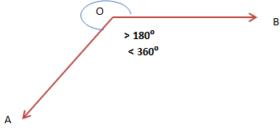Courses

# Important Questions Test: Basic Geometrical Ideas

## 20 Questions MCQ Test Mathematics (Maths) Class 6 | Important Questions Test: Basic Geometrical Ideas

Description
This mock test of Important Questions Test: Basic Geometrical Ideas for Class 6 helps you for every Class 6 entrance exam. This contains 20 Multiple Choice Questions for Class 6 Important Questions Test: Basic Geometrical Ideas (mcq) to study with solutions a complete question bank. The solved questions answers in this Important Questions Test: Basic Geometrical Ideas quiz give you a good mix of easy questions and tough questions. Class 6 students definitely take this Important Questions Test: Basic Geometrical Ideas exercise for a better result in the exam. You can find other Important Questions Test: Basic Geometrical Ideas extra questions, long questions & short questions for Class 6 on EduRev as well by searching above.
QUESTION: 1

Solution:
QUESTION: 2

### An angle is made up of two rays starting from common end point.

Solution:

Angles are made when corners are formed. When two rays have a common end point, then two rays together are said to form an angle. An angle is made up of two rays starting from a common end point. The two rays forming the angle are called the arms or sides of the angle. The common end point is the vertex of the angle.

QUESTION: 3

### Number of lines which can be drawn from one point:

Solution:
QUESTION: 4

Angle which is less than 360° and larger than 180° is classified as

Solution:

An angle which measures greater than 180 degrees but less than 360 degrees is known as a reflex angle.QUESTION: 5

A flat surface which extends indefinitely in all directions is called ___________ .

Solution:
QUESTION: 6

A __________ of a circle is a line segment joining any two points on the circle.

Solution:

chord of a circle is a straight line segment whose endpoints both lie on the circle. The infinite line extension of a chord is a secant line, or just secant. More generally, a chord is a line segment joining two points on any curve, for instance, an ellipse. The word chord is from the Latin chorda meaning bowstring.

QUESTION: 7

Angle which is equal to 90° is classified as

Solution:
QUESTION: 8

If line segment is extended in two directions indefinitely from each of two points then it is classified as

Solution:
QUESTION: 9

Flat surface in which two points are joined by using straight line is classified as

Solution:

Solution :- Flat surface in which two points are joined by using straight line is classified as plane.

QUESTION: 10

Any line segment can be formed by joining

Solution:
QUESTION: 11

Out of following, one angle which is obtuse is

Solution:

8/20 x 360 = 8 x 18 = 144 degrees which is more than 90 but less than 180

QUESTION: 12

If two lines intersects each other then the common point between them is known as point of _________.

Solution:

Two lines intersect when they cross each other. They form vertically opposite angles, which we will learn later. The point where the lines intersect is called the point of intersection.

QUESTION: 13

Two lines meeting at a point are called ___________ .

Solution:
QUESTION: 14

The edge of a ruler draws _____________ .

Solution:

a ruler draws line segment.

QUESTION: 15

Angle which is less than 90° is called

Solution:

Acute angles measure less than 90 degrees

QUESTION: 16

Three or more points lying on the same line are known as ___________ points.

Solution:
QUESTION: 17

Out of following options, two angles that are together classified as complementary angles are

Solution:
QUESTION: 18

When two rays meet each other at a certain point then the

Solution:
QUESTION: 19

Two lines in a plane either intersect exactly at one point or are

Solution: